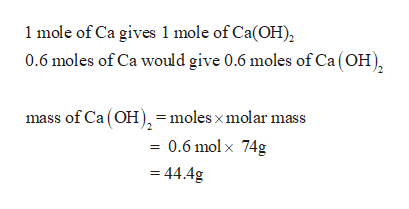# When 24.0 g of calcium metal is reacted with water, 5.00 g of calcium hydroxide is produced. Using the following balanced equation, calculate the percent yield for the reaction.Ca(s) + 2 H2O(l) → Ca(OH)2(aq) + H2(g)

Question
48 views

When 24.0 g of calcium metal is reacted with water, 5.00 g of calcium hydroxide is produced. Using the following balanced equation, calculate the percent yield for the reaction.

Ca(s) + 2 H2O(l) → Ca(OH)2(aq) + H2(g)

check_circle

Step 1

Given data

Mass of calcium = 24.0g

Mass of calcium hydroxide= 5.0g

Step 2

Moles of Calcium

Step 3

According to the...help_outlineImage Transcriptionclose1 mole of Ca gives 1 mole of Ca(OH) 0.6 moles of Ca would give 0.6 moles of Ca (OH) mass of Ca (OHmoles x molar mass = 0.6 mol x 74g 44.4g fullscreen

### Want to see the full answer?

See Solution

#### Want to see this answer and more?

Solutions are written by subject experts who are available 24/7. Questions are typically answered within 1 hour.*

See Solution
*Response times may vary by subject and question.
Tagged in

### Chemistry